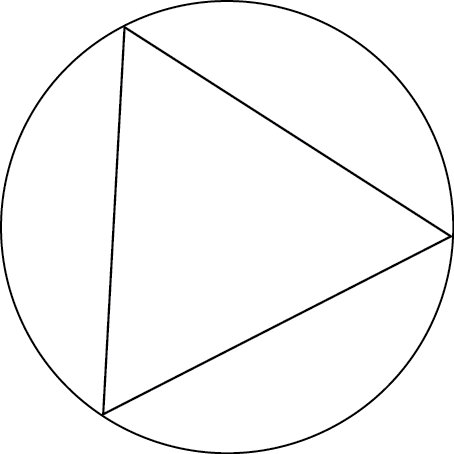## ________________

One summer project for the team is connecting with counterparts abroad to swap training problems. Here are a couple of favorites. (Thanks to the ELTE Matematikai Múzeum in Budapest for suggesting these problems.)

## ________________### Circular Triangle

Equilateral triangle ABC is inscribed in circle O. Chord DG of circle O intersects the triangle at E and F, with E nearer to D. Lines AE and BF are the angle bisectors of angles A and B of the triangle.

If the length of segment DE is 2, what is the length of segment EF ?

### Spherical Cube

A cube with unit edge length is inscribed in a sphere. Points C and D are the centers of adjacent faces of the cube. Ray r begins at C and passes through D.

What is the distance from D to the point where ray r intersects the sphere?

## Solutions to week 47

How Many. First, imagine the nine straight cuts did not intersect each other at all. They would clearly divide the cake into ten pieces. Now, notice that as you alter the cuts and angle one so that it intersects another, it creates another piece where they intersect. So there is one additional piece for each intersection, for a total of 17 pieces in all (and hence 17 people celebrating their birthdays).

Coach Newton elaborates:
If that solution didn’t seem quite rigorous enough to you, you can also use Euler’s Formula. There are 18 places where the cuts intersect the perimeter, and seven more places where they intersect each other, for 25 vertices. And there are 18 segments of the perimeter of the cake, the 9 cuts, and 14 more segments created from the seven intersections (every time two cuts intersect each other they create two new segments), for a total of 41 edges. Hence the formula VE + F = 2 tells you that F = 2 + 41 – 25 = 18, but one of the “faces” is the everything outside of the cake, so there are 17 pieces in all.

How Old. First, we know from one sibling having started high school no more than two years early and the oldest sibling having not yet been eligible to vote in a general election that we are looking for a number that has a factorization into three factors with largest factor 16, 17, or 18. But we also know the factorization of that product with those properties cannot be unique, because if it were, then Cameron would not need more clues to figure out the ages. Therefore, one of the other ages must be composite. Finally, how could the last clue provide Cameron with any additional information? It simply tells us the three siblings birthdays come a month apart. But that means that the three siblings are different ages. Hence, we need a factorization into three factors (with largest equal to 16, 17, or 18) such that the only other factorization into three factors (with largest equal to 16, 17, or 18) has two of the factors the same. Then when the possibility of twins is eliminated by the last clue, Cameron knows all of the ages.

Given that there must be a factorization with two factors the same, we are looking for a product of ages divisible by a square, with a factorization with largest factor 16, 17, or 18. There appear to be two possibilities: 1,536 = 16 × 16 × 6 = 16 × 12 × 8 and 1,088 = 17 × 8 × 8 = 17 × 16 × 4. However, even before the last clue, Cameron hears about “the one oldest sibling”, indicating that the oldest sibling is not a twin. So the only ambiguity that can remain before the last clue is that the younger siblings might be twins. Hence, the product is 1,088, and the three siblings are 17, 16, and 4 years old.

## Recent Weeks

Links to all of the puzzles and solutions are on the Complete Varsity Math page.

Come back next week for answers and more puzzles.

[asciimathsf]